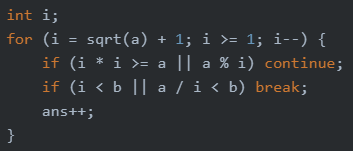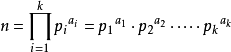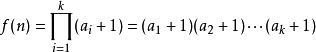• 前置知识——因数个数定理 描述： 将一个数xxx质因数分解后，可以表示为 x=p1c1p2c2...pkckx=p_1^{c_1}p_2^{c_2}...p_k^{c_k}x=p1c1​​p2c2​​...pkck​​，那么xxx的因数个数就是 ∏i=1k(ci+1)\prod\limits_{i=1}...
题目传送门
题目大意： 若

x

x

可以分解成

p

1

k

1

p

2

k

2

p

3

k

3

.

.

.

p

s

k

s

p_1^{k_1}p_2^{k_2}p_3^{k_3}...p_s^{k_s}

，那么

f

(

x

)

=

(

k

1

+

1

)

(

k

2

+

1

)

.

.

.

(

k

s

+

1

)

f(x)=(k_1+1)(k_2+1)...(k_s+1)

，求出

∑

i

=

l

r

f

(

i

)

\sum_{i=l}^r f(i)

前置知识——因数个数定理
描述： 将一个数

x

x

质因数分解后，可以表示为

x

=

p

1

c

1

p

2

c

2

.

.

.

p

k

c

k

x=p_1^{c_1}p_2^{c_2}...p_k^{c_k}

，那么

x

x

的因数个数就是

∏

i

=

1

k

(

c

i

+

1

)

\prod\limits_{i=1}^k(c_i+1)

。
证明： 由于

x

x

的每个因子也可以表示上面的形式，只不过

c

c

的值不同，设因子的质因数分解后为

p

1

c

1

′

p

2

c

2

′

.

.

.

p

k

c

k

′

p_1^{c_1'}p_2^{c_2'}...p_k^{c_k'}

，对于

c

i

′

c_i'

，它的取值范围是

0

0

~

c

i

c_i

，即有

(

c

i

+

1

)

(c_i+1)

种取值方案，所以总因子个数就是

∏

i

=

1

k

(

c

i

+

1

)

\prod\limits_{i=1}^k(c_i+1)

。
题解
发现题目定义的

f

(

i

)

f(i)

其实就是

i

i

的因数个数。
那么考虑换一个角度，统计每一个因数

x

x

会被多少个

i

i

计算到。
显然，

x

x

的每个倍数都会将

x

x

计算一次，将询问差分一下，变成

∑

i

=

1

r

f

(

i

)

−

∑

i

=

1

l

−

1

f

(

i

)

\sum_{i=1}^rf(i)-\sum_{i=1}^{l-1}f(i)

，那么问题就变成了求

1

1

~

n

n

范围内有多少个

x

x

的倍数，即

求

∑

i

=

1

n

⌊

n

i

⌋

\sum_{i=1}^n \lfloor \frac n i \rfloor

然而这是个除法分块的模板。
代码如下：
#include <cstdio>
#include <cstring>
#define mod 998244353ll
#define ll long long

ll a,b;
ll solve(ll x)
{
ll l=1,r,re=0;
while(l<=x)
{
r=x/(x/l);
re=(re+(r-l+1)*(x/l)%mod)%mod;
l=r+1;
}
return re;
}

int main()
{
scanf("%lld %lld",&a,&b);
printf("%lld",(solve(b)-solve(a-1)+mod)%mod);
}

展开全文• 题解：唯一分解定理求a的因数个数，然后除2取整（懂的都懂，另外如果存在sqrt(a)*sqrt(a)=a，也是这样，不用特意考虑，所以count部分完全模板）。然后cnt暴力计数小于b的是否a%i==0（这里注意可能3*4=a=12,b=5，会...
传送门

题意：给两个数a,b，a为某个长方形的面积（不能为正方形），求长和宽都不小于b且面积为a的长方形的个数。
1 ≤ b ≤ a ≤ 1e12多组输入T<=4000

题解：唯一分解定理求a的因数个数，然后除2取整（懂的都懂，另外如果存在sqrt(a)*sqrt(a)=a，也是这样，不用特意考虑，所以count部分完全模板）。然后cnt暴力计数小于b的数是否a%i==0（这里注意可能3*4=a=12,b=5，会记录两次，但是不用考虑，可以直接特判b*b>=a 返回答案0，懂的都懂）。
答案为count(x)/2-cnt。
复杂度最高O(4e9)勉强过了（感觉数论都是这种最高1e9+，但是一般都不会达到。忽悠人？建议循环变量外置）。

ps：听别人说唯一分解定理之后可以快速求出这个数的所有因数？？？反正网上没人写这个代码的样子。

代码：
#include <bits/stdc++.h>
#define int long long
#define print(a, c) printf("%lld%c", a, c)
#define dbg(x) cout << #x << "===" << x << endl
using namespace std;
const int N = 1e6 + 10;

int a, b;

int prime[N], vis[N];
void init(int n) {
for (int i = 2; i <= n; i++) {
if (vis[i]) continue;  //非素数
prime[++prime] = i;
for (int j = i + i; j <= n; j += i) vis[j] = 1;
}
}
//先素数筛算是一个优化，大概能优化一个数量级吧
int count(int x) {
int res = 1, i, a;
//从1开始，prime为素数个数
//不能等于，因为不能为正方形
//不过，尽管大胆一点去分解，若存在sqrt(x)*sqrt(x)=x，无非因数个数为奇数
for (i = 1; prime[i] * prime[i] <= x && i <= prime; i++) {
a = 0;
while (x % prime[i] == 0) a++, x /= prime[i];
res *= (a + 1);  //很多*1
}
if (x > 1) res *= (1 + 1);
return res;
}
signed main() {
init(N - 1);
int T;

int ans, cnt, i;
for (int _ = 1; _ <= T; _++) {
ans = 0;
if (b * b >= a)
ans = 0;
else {
cnt = 0, i;
for (i = 1; i < b; i++)
if (a % i == 0) cnt++;
ans = count(a) / 2 - cnt;
}
printf("Case %lld: %lld\n", _, ans);
}
return 0;
}

补充：蜜汁复杂度，如果严格按照要求的最大限制，那么上面的做法和下面暴力差不多吧。这题应该只是卡了下面这种做法。事实上应该可以互补，比如b太大就下面这种，太小就上面这种。感觉也优化不到什么emm展开全文线性筛
• 分析：唯一分解定理： 任何一个数都可以分解为合数素数，合数又可以分解为素数，所以素数打好表分解素数，利用定理即可。 AC代码： #include #include long long f,vis,k=0; int prime() { ...
我是传送门 It’s said that Aladdin had to solve seven mysteries before getting the Magical Lamp which summons a powerful Genie. Here we are concerned about the first mystery. Aladdin was about to enter to a magical cave, led by the evil sorcerer who disguised himself as Aladdin’s uncle, found a strange magical flying carpet at the entrance. There were some strange creatures guarding the entrance of the cave. Aladdin could run, but he knew that there was a high chance of getting caught. So, he decided to use the magical flying carpet. The carpet was rectangular shaped, but not square shaped. Aladdin took the carpet and with the help of it he passed the entrance. Now you are given the area of the carpet and the length of the minimum possible side of the carpet, your task is to find how many types of carpets are possible. For example, the area of the carpet 12, and the minimum possible side of the carpet is 2, then there can be two types of carpets and their sides are: {2, 6} and {3, 4}. Input Input starts with an integer T (≤ 4000), denoting the number of test cases. Each case starts with a line containing two integers: a b (1 ≤ b ≤ a ≤ 1012) where a denotes the area of the carpet and b denotes the minimum possible side of the carpet. Output For each case, print the case number and the number of possible carpets. 1325/5000 据说阿拉丁在得到神灯之前必须解决七个谜题，神灯会召唤出一个强大的精灵。这里我们关注的是第一个谜团。
阿拉丁正要进入一个神奇的山洞，由一个化装成阿拉丁叔叔的邪恶巫师带领，在洞口发现了一块奇怪的魔法飞毯。有一些奇怪的生物守卫着洞穴的入口。阿拉丁可以逃跑，但他知道被抓住的可能性很大。所以，他决定使用魔法飞毯。地毯是长方形的，但不是方形的。阿拉丁拿过地毯，搀着它穿过了入口。
现在你得到了地毯的面积和地毯可能的最小边的长度，你的任务是找出有多少种地毯是可能的。例如，地毯的面积12，地毯的最小可能边是2，那么可以有两种类型的地毯，它们的边是:{2,6}和{3,4}。
输入 输入以整数T(≤4000)开始，表示测试用例数。 每种情况都以包含两个整数的一行开始:a b(1≤b≤a≤1012)，其中a表示地毯的面积，b表示地毯的最小可能边。 输出 每箱打印箱号和可能使用的地毯数量。
题意如上很清楚。
分析：唯一分解定理：任何一个数都可以分解为合数和素数，合数又可以分解为素数，所以素数打好表分解素数，利用定理即可。
AC代码：
#include<stdio.h>
#include<string.h>
long long f,vis,k=0;
int prime()
{
int i,j;
vis=1;//1必须先标记，循环从2开始
for(i=2;i<=1000010;i++)
{
if(!vis[i])
{
f[k++]=i;
for(j=i*2;j<=1000000;j+=i)
vis[j]=1;
}
}
}
int main()
{
long long g,t,n,m,nn,ans,b,i;
g=1;
scanf("%lld",&t);
prime();
while(t--)
{
scanf("%lld%lld",&n,&m);
long long p=m*m;
if(m*m>=n)//特殊情况，0个
{
printf("Case %lld: 0\n",g++);
continue;
}
else
{
nn=n;
b=1;
for(i=0;i<k&&f[i]*f[i]<=n;i++)
{
if(n%f[i]==0)
{
ans=0;
while(n%f[i]==0)//唯一分解定理主要部分
{
ans++;
n=n/f[i];
}
b=b*(ans+1);
}
}
for(int p=1;p<m;p++)//定理找的是所有正因数，题目给出了最小因数m，舍掉更小的
{
if(nn%p==0)
b--;
}
printf("Case %lld: %lld\n",g++,b);
}
}
}

展开全文• 约数个数定理 则n的正约数的个数就是 。 其中a1、a2、a3…ak是p1、p2、p3，…pk的指数。 定理简证 编辑 首先同上，n可以分解质因数：n=p1^a1×p2^a2×p3^a3*…*pk^ak, 由约数定义可知p1^a1的约数有:p1^0, ...
整数的唯一分解定理
对于一个大于1正整数n可以分解质因数：约数个数定理
则n的正约数的个数就是。
其中a1、a2、a3…ak是p1、p2、p3，…pk的指数。
定理简证
编辑
首先同上，n可以分解质因数：n=p1^a1×p2^a2×p3^a3*…*pk^ak,
由约数定义可知p1^a1的约数有:p1^0, p1^1, p1^2......p1^a1 ，共（a1+1）个;同理p2^a2的约数有（a2+1）个......pk^ak的约数有（ak+1）个。
故根据乘法原理：n的约数的个数就是(a1+1)(a2+1)(a3+1)…(ak+1)。
乘法原理
做一件事，完成它需要分成n个步骤，做第一 步有m1种不同的方法，做第二步有m2种不同的方法，……，做第n步有mn种不同的方法。那么完成这件事共有 N=m1×m2×m3×…×mn 种不同的方法。
例题链接:UVA-294https://vjudge.net/problem/UVA-294
约数和公式
对于已经分解的整数A=(p1^k1)*(p2^k2)*(p3^k3)*....*(pn^kn)
有A的所有因子之和为
S = (1+p1+p1^2+p1^3+...p1^k1) * (1+p2+p2^2+p2^3+….p2^k2) * (1+p3+ p3^3+…+ p3^k3) * .... * (1+pn+pn^2+pn^3+...pn^kn)  
展开全文• LightOJ - 1341 Aladdin and the Flying Carpet (唯一分解定理) 题意：给一 长方形 的面积a，求有多少组整数边...我们可以知道一个数可以分解成若干质数相乘，并且可能会有一些相同的质数在里面，表示为 x=p1a1+
• 知识点 - 因数 因数个数公式 解决问题类型： 问有几个因数因数， 或者问某些特定约数之，比如不能被大于4的平方整除的约数之（即质因数的次数都为1） 结论 若对 nnn 质因数分解得到 p1e1⋅p2e2⋯...
• 利用线性筛算法框架求解因数个数以及因数和问题 前言 关于线性筛算法，在前一篇文章
• 给一对数字 a，b ，a是一长方形的面积，问有多少种整数的边的组合可以组成面积为a的长方形，要求最短的边不得小于b Input Input starts with an integer T (≤ 4000), denoting the number of test cases. E...
• 本文主要讲解如何判断一个数是质数，如何对一个数分解质因数。本文是很基础的也很重要的数学知识。 质数 质数又称为素数，是指大于1的并且除了1它本身外，没有其他因数的自然数。 判断一个数是否是质数 假设该...
• 换句话说，一个数能被唯一地分解成质因数的乘积。 公式：， 因子： p1可以取的个数为[0, a1]，p2可以取的个数为[0, a2]，pk可以取的个数为[0, ak]，根据乘法原理，总的因子个数就是这些指数+1的连乘，即(1 + ...
•   我们知道，素数ppp（p≥2p \ge 2p≥2）是正因数仅有111ppp的，不是素数的整数mmm（m≥2m\ge 2m≥2）叫做合数： 素数:2,3,5,7,11,13,17,19,23,29,31,⋯合数:4,6,8,9,10,12,14,15,16,18,20,⋯ 素数:2,3,5,7,11...
• 性质： 一、对于一大于1的正整数 n 可以分解质因数：n=p1^a1 * ...二、约数个数定理 n的正约数有(a₁+1)(a₂+1)(a₃+1)…(ak+1) 对于12来说 就是（2+1）*（1+1）=6 有6约数 1 2 3 4 6 12 三、约数和定理 f(...
• 唯一分解定理:任何一大于1的自然数N,如果N不为质数，那么N可以唯一分解成有限质数的乘积...分解质因数，一个数n的质因数只能全部小于等于sqrt(n),或者只有一大于sqrt(n) map<int,long long>q; fo...
• 暴力求 n 的约数个数、约数的复杂度为 ，往往不能符合题目时限要求。但这通过质因数分解来优化时间。 质因数分解： 性质：一个数 n ，最多只存在一质因子。 因此，我们只需要预处理出以内的质数（1e5以内...
• 定理2（算数基本定理）：每整数n≥2可唯一分解成素数乘积 n=p1p2···pr 例如：12=2·2·312=3·2·2看成相同的因式分解。 练习： 编写将（正）整数n分解成素数乘积的程序。（如果n=0,确保转到出错信息而不是...c++
• ## 质因数分解定理

千次阅读 2013-12-03 18:53:26
概况：算术基本定理：“每一大于1的整数都能分解成质因数乘积的形式，并且如果把质因数按照由小到大的顺序排列在一起，相同的因数的积写成幂的形式，那么这种分解方法是唯一的。”——又称为“质因数分解定理”，...acm c++
• 约数个数定理 对于一大于1正整数n可以分解质因数：n=p1a1*p2a2*p3a3*…*pkak, 则n的正约数的个数就是（a1+1）（a2+1）（a3+1）…(ak+1) . ll getnum(ll n) //得到a的约数个数. { ll res=1; for(ll i=2;i*i<=n...
• 约数个数定理对于一大于1正整数n可以分解质因数： 则n的正约数的个数就是 。其中a1、a2、a3…ak是p1、p2、p3，…pk的指数。约数定理证明首先同上，n可以分解质因数：n=p1^a1×p2^a2×p3^a3*…*pk^ak,由约数定义...
• 因数分解（唯一分解定理） 基本概念： 每合数都可以写成几质数相乘的形式，其中每质数都是这合数的因数，叫做这合数的分解质因数。 分解质因数只针对合数。 并且，每合数能够且仅仅能够被分解为唯...
• 1、如果我们要求一个数的所有因数的个数会怎么去求呢？ 首先想到最简单的方法就是暴力求解就可以。当然数据小、或者测试数据少就很简单就可以过了。 2、如果求一区间内的的所有因数的个数呢？或者求一区间内...
• 因数分解算数基本定理 素数是这样的整数p≥2p\geq2p≥2，它的（正）因数仅有1p。不是素数的整数m≥2m\geq2m≥2叫做合数。例如，素数2，3，5，7，11，13，⋯素数 2，3，5，7，11，13，\cdots素数2，3，5，7，11，...
• 唯一质因数分解定理: 任意一合数a仅能以一种方式，写成如下的乘积形式： $$a$$ =$p1^{e1}\times p2^{e2}\times ...\times pr^{er}$ $$a$$的因子= $$(e1+1)\times (e2+1)\times ....\times (er+1)$$ const int N......